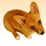# Python to compare values for ranking

908
5
05-29-2022 08:05 PMby
Occasional Contributor III

Hi All,

I have a dataset with four 'types' and their 'counts' for example:

A = 4, B = 2, C = 0, D = 0

In order of ranking, D (is the most important) > C > B > A (lowest ranking).

If I have an output with:

A = 0, B = 1, C = 0 , D = 1

How do I script in Python to compare the string so that when B = D that the variable I capture will be D based on ranking?

Regards,

Craig

1 Solution

Accepted SolutionsbyMVP Frequent Contributor
• what is the type of your output? string, list, dict, something else?
• are the types actually just letters or are you simplifying things?

Assuming that you return a string and that the types are just letters (getting more important in ascending alphabetical order):

``````output = "A = 0, B = 1, C = 0 , D = 1"

# change to [ [type, count] ]
converted_output = output.replace(" ", "").split(",")
converted_output = [tc.split("=") for tc in converted_output]
print(f"converted output: {converted_output}")

# sort by count and type (both descending), return first element
sorted_output = sorted(converted_output, key=lambda r: (r, r), reverse=True)
print(f"sorted output: {sorted_output}")
most_significant_output = sorted_output
print(f"most significant output: {most_significant_output}")

#converted output: [['A', '0'], ['B', '1'], ['C', '0'], ['D', '1']]
#sorted output: [['D', '1'], ['B', '1'], ['C', '0'], ['A', '0']]
#most significant output: ['D', '1']``````

If your types are actually not letters but e.g. species, you have to define how to rank them and then use the list.index(element) method in the sort:

``````output = "Pig = 1, Lamb = 1, Chicken = 0, Duck = 1, Cow = 0"

# specify the ranking of the types, starting from lowest
ranked_types = ["Cow", "Pig", "Duck", "Horse", "Lamb", "Chicken"]

# change to [ [type, count] ]
converted_output = output.replace(" ", "").split(",")
converted_output = [tc.split("=") for tc in converted_output]
print(f"converted output: {converted_output}")

# sort by count and type (both descending), return first element
sorted_output = sorted(converted_output, key=lambda r: (r, ranked_types.index(r)), reverse=True)
print(f"sorted output: {sorted_output}")
most_significant_output = sorted_output
print(f"most significant output: {most_significant_output}")

#converted output: [['Pig', '1'], ['Lamb', '1'], ['Chicken', '0'], ['Duck', '1'], ['Cow', '0']]
#sorted output: [['Lamb', '1'], ['Duck', '1'], ['Pig', '1'], ['Chicken', '0'], ['Cow', '0']]
#most significant output: ['Lamb', '1']``````

Have a great day!
Johannes
5 RepliesbyMVP Frequent Contributor
• what is the type of your output? string, list, dict, something else?
• are the types actually just letters or are you simplifying things?

Assuming that you return a string and that the types are just letters (getting more important in ascending alphabetical order):

``````output = "A = 0, B = 1, C = 0 , D = 1"

# change to [ [type, count] ]
converted_output = output.replace(" ", "").split(",")
converted_output = [tc.split("=") for tc in converted_output]
print(f"converted output: {converted_output}")

# sort by count and type (both descending), return first element
sorted_output = sorted(converted_output, key=lambda r: (r, r), reverse=True)
print(f"sorted output: {sorted_output}")
most_significant_output = sorted_output
print(f"most significant output: {most_significant_output}")

#converted output: [['A', '0'], ['B', '1'], ['C', '0'], ['D', '1']]
#sorted output: [['D', '1'], ['B', '1'], ['C', '0'], ['A', '0']]
#most significant output: ['D', '1']``````

If your types are actually not letters but e.g. species, you have to define how to rank them and then use the list.index(element) method in the sort:

``````output = "Pig = 1, Lamb = 1, Chicken = 0, Duck = 1, Cow = 0"

# specify the ranking of the types, starting from lowest
ranked_types = ["Cow", "Pig", "Duck", "Horse", "Lamb", "Chicken"]

# change to [ [type, count] ]
converted_output = output.replace(" ", "").split(",")
converted_output = [tc.split("=") for tc in converted_output]
print(f"converted output: {converted_output}")

# sort by count and type (both descending), return first element
sorted_output = sorted(converted_output, key=lambda r: (r, ranked_types.index(r)), reverse=True)
print(f"sorted output: {sorted_output}")
most_significant_output = sorted_output
print(f"most significant output: {most_significant_output}")

#converted output: [['Pig', '1'], ['Lamb', '1'], ['Chicken', '0'], ['Duck', '1'], ['Cow', '0']]
#sorted output: [['Lamb', '1'], ['Duck', '1'], ['Pig', '1'], ['Chicken', '0'], ['Cow', '0']]
#most significant output: ['Lamb', '1']``````

Have a great day!
Johannesby
Occasional Contributor III

Your script interprets exactly what I am after, but I have one final issue.

My list of tuple values 'lst' works for the majority of returned values, however I occasionally have the instance where the value sorted and ranked is incorrect such as below:

lst = [['Rabbit', '7'], ['Dog', '3'], ['Bird', '17'], ['Cat', '0']]
rnk_lst = ['Cat', 'Bird', 'Dog', 'Rabbit']
sorted_output = sorted(lst, key=lambda r: (r, rnk_lst.index(r)), reverse=True)
print(f"sorted output: {sorted_output}")

most_significant_output = sorted_output
print(most_significant_output)

```sorted output: [['Rabbit', '7'], ['Dog', '3'], ['Bird', '17'], ['Cat', '0']]
Rabbit```

If the maximum value is ['Bird', 17] that is the value I expected, yet script equates Rabbit.

Not sure why it works for most of analysis process, but trips up on a few response. Any ideas?

CraigbyMVP Frequent Contributor

It's because Python sorts the counts as strings (because they are), not as numbers. And in string sorting, "7" is greater than "17".

To solve that, you have to cast the string to int in the sorted() call.

``````lst = [['Rabbit', '7'], ['Dog', '3'], ['Bird', '17'], ['Cat', '0']]
rnk_lst = ['Cat', 'Bird', 'Dog', 'Rabbit']
sorted_output = sorted(lst, key=lambda r: (int(r), rnk_lst.index(r)), reverse=True)
print(f"sorted output: {sorted_output}")

most_significant_output = sorted_output
print(most_significant_output)``````

```sorted output: [['Bird', '17'], ['Rabbit', '7'], ['Dog', '3'], ['Cat', '0']]
Bird```

Have a great day!
Johannesby
Occasional Contributor III

That solves my issue perfectly. Thank you.byMVP Esteemed Contributor

You are asking about the situation when 2 of the types have the same value, but it is hard for people to provide any code samples when they don't know what is supposed to be returned in general.  Are you trying to return the type with the highest count from the data?  And when the highest count is shared between types, you want only the most important type?